##### Basic Math & Pre-Algebra All-in-One For Dummies (+ Chapter Quizzes Online)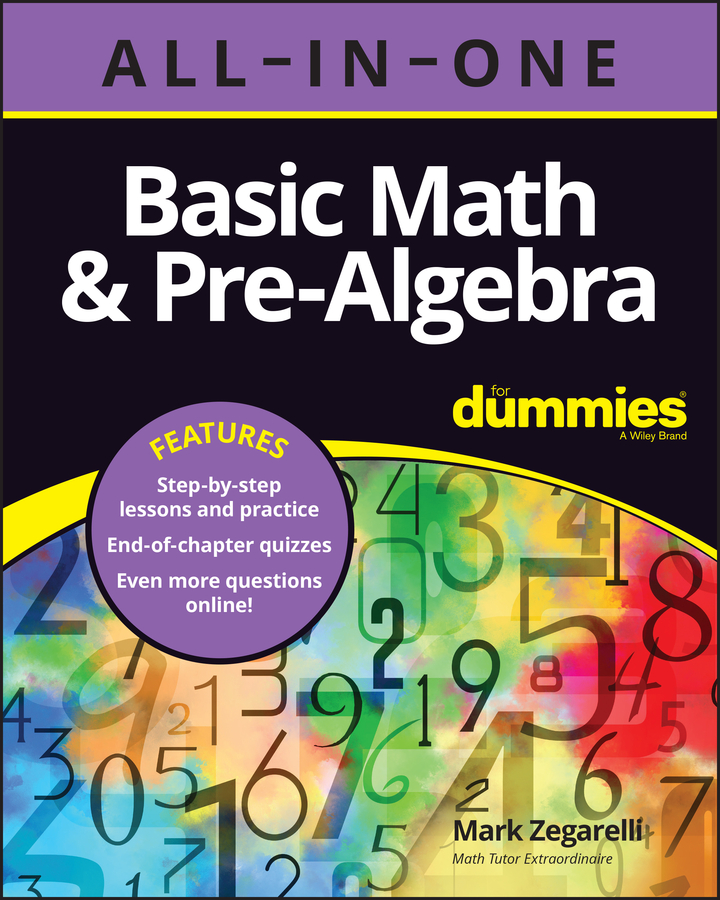Whether mixed numbers have the same denominators or different denominators, subtracting them is a lot like subtracting whole numbers: you stack them up one on top of the other, draw a line, and subtract. For this reason, some students feel more comfortable subtracting mixed numbers than subtracting fractions.

1. Find the difference of the fractional parts.

2. Find the difference of the two whole number parts.

Along the way, though, you may encounter a couple more twists and turns. The following instructions will keep you on track so that you can do any mixed-number subtraction problem.

## Subtract mixed numbers with the same denominators

Subtraction is much easier when the denominators are the same. For example, suppose you want to subtract 7 3/5 – 3 1/5. Here’s what the problem looks like in column form: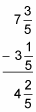In this problem, you subtract 3/5 – 1/5 = 2/5. Then you subtract 7 – 3 = 4. Not too terrible, agreed?

One complication arises when you try to subtract a larger fractional part from a smaller one. Suppose you want to find 11 1/6 – 2 5/6. This time, if you try to subtract the fractions, you get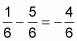Obviously, you don’t want to end up with a negative number in your answer. You can handle this problem by borrowing from the column to the left. This idea is very similar to the borrowing that you use in regular subtraction, with one key difference.

When borrowing in mixed-number subtraction,

1. Borrow 1 from the whole-number portion and add it to the fractional portion, turning the fraction into a mixed number.

To find 11 1/6 – 2 5/6, borrow 1 from the 11 and add it to 1/6, making it the mixed number 1 1/6:

2. Change this new mixed number into an improper fraction.

Here’s what you get when you change 1 1/6 into an improper fraction: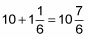The result is 10 7/6. This answer is a weird cross between a mixed number and an improper fraction, but it’s what you need to handle the job.

3. Use the result in your subtraction.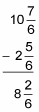In this case, you have to reduce the fractional part of the answer: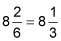## Subtract mixed numbers with different denominators

Subtracting mixed numbers when the denominators are different is just about the hairiest thing you’re ever going to have to do in pre-algebra. Suppose you want to subtract 15 4/11 – 12 3/7. Because the denominators are different, subtracting the fractions becomes more difficult. But you have another question to think about: In this problem, do you need to borrow? If 4/11 is greater than 3/7, you don’t have to borrow. But if 4/11 is less than 3/7, you do.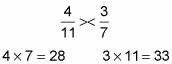Because 28 is less than 33, 4/11 is less than 3/7, so you do have to borrow. Get the borrowing out of the way first: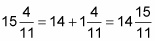Now the problem looks like this: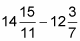The first step, subtracting the fractions, is going to be the most time-consuming, so you can take care of that on the side: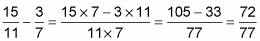The good news is that this fraction can’t be reduced. (They can’t be reduced because 72 and 77 have no common factors: 72 = 2 2 2 3 3, and 77 = 7 11.) So the hard part of the problem is done, and the rest follows easily: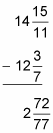This problem is about as difficult as a mixed-number subtraction problem gets. Take a look over it step by step. Or better yet, copy the problem and then try to work through the steps on your own. If you get stuck, that’s okay. Better now than on an exam!